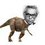# Intriguing problem

Today, I , Svatejas and Vighnesh were chatting on slack and I accidentally came across this problem:

Find the largest integer $n$ such that $n! for some positive integer $a$ having $n$ digits.

Find a method to do this. Hoping for numerous approaches :)Note by Nihar Mahajan
5 years, 5 months ago

This discussion board is a place to discuss our Daily Challenges and the math and science related to those challenges. Explanations are more than just a solution — they should explain the steps and thinking strategies that you used to obtain the solution. Comments should further the discussion of math and science.

When posting on Brilliant:

• Use the emojis to react to an explanation, whether you're congratulating a job well done , or just really confused .
• Ask specific questions about the challenge or the steps in somebody's explanation. Well-posed questions can add a lot to the discussion, but posting "I don't understand!" doesn't help anyone.
• Try to contribute something new to the discussion, whether it is an extension, generalization or other idea related to the challenge.

MarkdownAppears as
*italics* or _italics_ italics
**bold** or __bold__ bold
- bulleted- list
• bulleted
• list
1. numbered2. list
1. numbered
2. list
Note: you must add a full line of space before and after lists for them to show up correctly
paragraph 1paragraph 2

paragraph 1

paragraph 2

[example link](https://brilliant.org)example link
> This is a quote
This is a quote
    # I indented these lines
# 4 spaces, and now they show
# up as a code block.

print "hello world"
# I indented these lines
# 4 spaces, and now they show
# up as a code block.

print "hello world"
MathAppears as
Remember to wrap math in $$ ... $$ or $ ... $ to ensure proper formatting.
2 \times 3 $2 \times 3$
2^{34} $2^{34}$
a_{i-1} $a_{i-1}$
\frac{2}{3} $\frac{2}{3}$
\sqrt{2} $\sqrt{2}$
\sum_{i=1}^3 $\sum_{i=1}^3$
\sin \theta $\sin \theta$
\boxed{123} $\boxed{123}$

Sort by:

I'll try to list all such integer till n=6 by tmmrw.(through coding obviously)

- 5 years, 5 months ago

Here is an interesting result: $\lceil \log([\log(2^{10000})]!) \rceil = 9166$

- 5 years, 5 months ago

That was indeed surprising LOL

- 5 years, 5 months ago

@Nihar Mahajan Let's create some problem using factorials and 9166 tomorrow. Till then I won't follow anyone :P

- 5 years, 5 months ago

Sure!

- 5 years, 5 months ago

- 5 years, 5 months ago

There is no one-formula for this question.

- 5 years, 5 months ago

If we include some relation between $a$ and $n$ then? For example: if $a$ has $n$ digits.

- 5 years, 5 months ago

Then you can use Stirling's formula.

- 5 years, 5 months ago